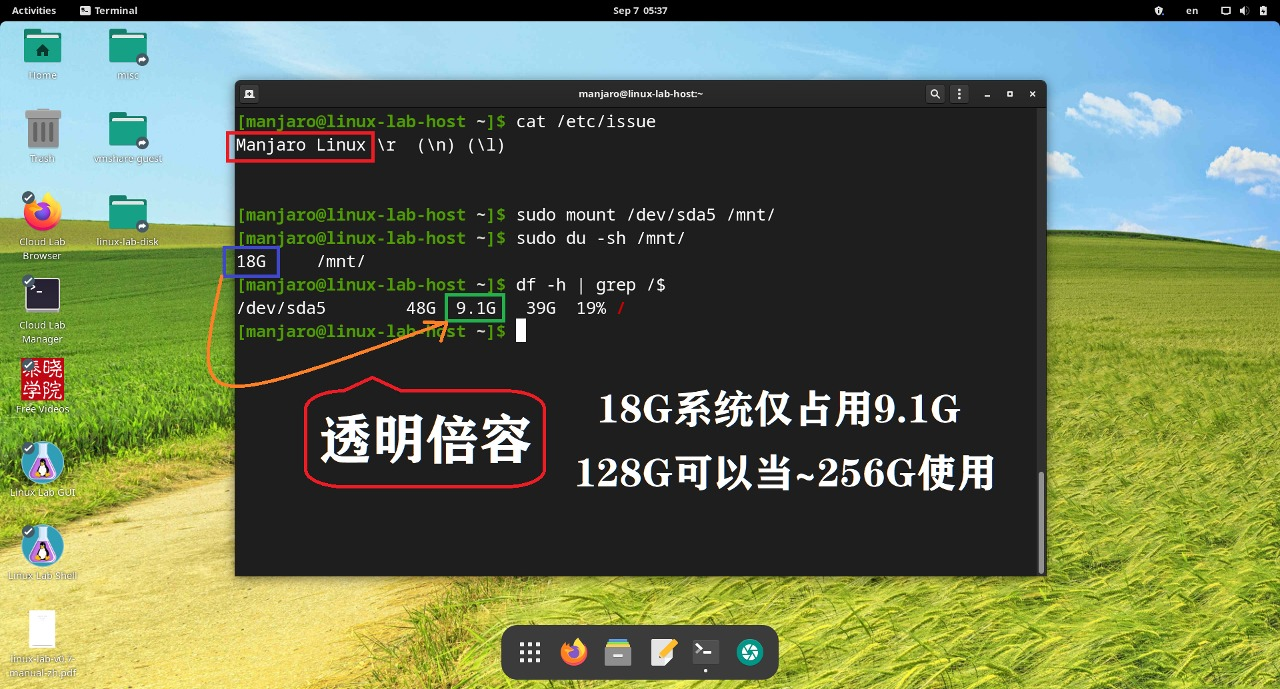# Shell 编程学习笔记

by falcon of TinyLab.org 2006/04/03

## 执行 Shell 脚本的方式

### 范例：输入重定向到 Bash

``````\$ bash < ex1
``````

### 范例：以脚本名作为参数

``````\$ bash 脚本名 [参数]
``````

``````\$ bash ex2 /usr/meng /usr/zhang
``````

### 范例：以 . 来执行

``````\$ · ex3[参数]
``````

### 范例：直接执行

``````\$ chmod a+x ex4
\$ ./ex4
``````

``````#!/bin/bash
``````

## Shell 的执行原理

Shell 接收用户输入的命令（脚本名），并进行分析。如果文件被标记为可执行，但不是被编译过的程序， Shell 就认为它是一个 Shell 脚本。 Shell 将读取其中的内容，并加以解释执行。所以，从用户的观点看，执行 Shell 脚本的方式与执行一般的可执行文件的方式相似。

## 变量赋值

### 范例：获取当前的工作目录并存放到变量中

``````\$ dir=`pwd`
``````

``````\$ dir=\$(pwd)
``````

## 数组

Bash 只提供一维数组，并且没有限定数组的大小。类似与 C 语言，数组元素的下标由 0 开始编号。获取数组中的元素要利用下标。下标可以是整数或算术表达式，其值应大于或等于 0 。用户可以使用赋值语句对数组变量赋值。

### 范例：对数组元素赋值

``````\$ city=Beijing
\$ city=Shanghai
\$ city=Tianjin
``````

``````\$ declare -a 数组名
``````

### 范例：访问某个数组元素

``````\$ echo \${city}
Beijing
``````

### 范例：数组组合赋值

``````数组名=(值1 值2 ... 值n)
``````

``````\$ A=(this is an example of shell script)
\$ echo \${A} \${A} \${A} \${A}
this an example script
\$ echo \${A}
``````

### 范例：列出数组中所有内容

``````\$ echo \${A[*]}
this is an example of shell script
``````

### 范例：获取数组元素个数

``````\$ echo \${#A[*]}
7
``````

## 参数传递

``````\$ cat > add
let sum=\$1+\$2
echo \$sum
``````

``````\$ chmod a+x ./add
15
``````

``````let sum=\$X+\$Y
echo \$sum
``````

``````\$ X=5 Y=10 ./add
15
``````

## 设置环境变量

export 一个环境变量：

``````\$ export opid=True
``````

## 键盘读起变量值

``````\$ read -p "请输入一个值 ： "  input ; echo "你输入了一个值为 ：" \$input

``````

## 设置变量的只读属性

``````\$ oracle_home=/usr/oracle7/bin
``````

## 条件测试命令 test

### 范例：数值比较

`-eg =；-ne !=；-gt >；-ge >=；-lt <；-le <=`

``````\$ test var1 -gt var2
``````

### 范例：测试文件属性

`-r; -w; -x; -f; -d`

``````\$ test -r filename
``````

### 范例：字符传属性以及比较

``````\$ test -z s1
``````

### 范例：串比较

``````\$ if [[ "abcde" < "abcdf" ]]; then  echo "yeah,果然是诶"; fi
yeah,果然是诶
``````

## 整数算术或关系运算 expr

``````\$ i=5;expr \$i+5
``````

## 控制执行流程命令

### 范例：条件分支命令if

if 命令举例：如果第一个参数是一个普通文件名，那么分页打印该文件；否则，如果它为目录名，则进入该目录并打印该目录下的所有文件，如果也不是目录，那么提示相关信息。

``````if test -f \$1
then
pr \$1>/dev/lp0
elif
test-d \$1
then
(cd \$1;pr *>/dev/lp0)
else
echo \$1 is neither a file nor a directory
fi
``````

### 范例：case 命令举例

case 命令是一个基于模式匹配的多路分支命令，下面将根据用户键盘输入情况决定下一步将执行那一组命令。

``````while [ \$reply!="y" ] && [ \$reply!="Y" ]                         #下面将学习的循环语句
do
echo "\nAre you want to continue?(Y/N)\c"
case \$replay in
(y|Y) break;;         #退出循环
(n|N) echo "\n\nTerminating\n"
exit 0;;
continue;       #直接返回内层循环开始出继续
esac
done
``````

### 范例：循环语句 while, until

``````while/until 命令表1
do
命令表2
done
``````

### 范例：有限循环命令 for

``````for 变量名 in 字符串表
do
命令表
done
``````

``````FILE="test1.c myfile1.f pccn.h"
for i in \$FILE
do
cd ./tmp
cp \$i \$i.old
echo "\$i copied"
done
``````

## 函数

``````\$ cat > show
# 函数定义
function show
{
echo \$1\$2;
}
H="Hello,"
W="World!"
# 调用函数，并传给两个参数H和W
show \$H \$W
``````

``````\$ chmod 770 show
\$./show
Hello,World!
``````

``````\$ show \$H \$W
``````

``````function show
{
echo \$1
}
HW="Hello World"
show "\$HW"
``````